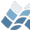NV5 GEOSPATIAL SOFTWARE## Purpose

This function returns an array with extra dimensions added, filled
with copies of the original array.

Array

## Calling Sequence

Result = add_dim( Array, Id_dim_new, Len_dim_new )

## Inputs

Array: An array of any type and number of dimensions.
Id_dim_new: The index number indicating where to add the new
dimensions. For instance, if Array=fltarr(4,5) and
Len_dim_new=3, then if Id_dim_new=0 then Result=fltarr(3,4,5),
if Id_dim_new=1 then Result=fltarr(4,3,5), and if Id_dim_new=2
then Result=fltarr(4,5,3).
Len_dim_new: The size of the new dimensions to add. This can be a
scalar or vector.

## Outputs

Result: The expanded array.

## Procedure

This function creates a new array and iteratively copies the old
array into the new array.

## Example

Define a 4*5-element array.
x = findgen( 4, 5 )
Add another dimension, of size 3, in the middle of the array.
result = add_dim( x, 1, 3 )
The function should return a 4*3*5 element array with entries
result[*,i,*] equal to reform( x, 4, 1, 5 ) for all i={0,1,2}.

## Modification History

Written by: Daithi A. Stone, 2007-08-01.
Modified: DAS, 2007-12-18 (changed to allow larger arrays
requiring long integer indices)
Modified: DAS, 2008-03-18 (completed previous modification)
Modified: DAS, 2009-03-31 (fixed bug in determining size and type
of Array)

© 2023 NV5 Geospatial |  Legal